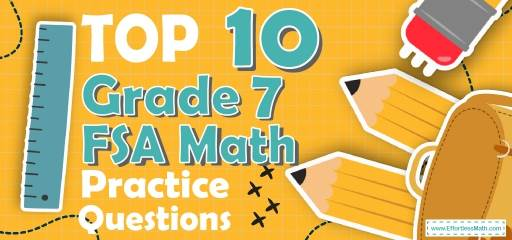# Top 10 7th Grade FSA Math Practice QuestionsThe best way to prepare your students for the 7th Grade FSA Math test is to work through as many FSA Math practice questions as possible. Here are the top 10 7th Grade FSA Math practice questions to help students review the most important 7th Grade FSA Math concepts. These 7th Grade FSA Math practice questions are designed to cover mathematics concepts and topics that are found on the actual test. The questions have been fully updated to reflect the latest 2022 7th Grade FSA guidelines. Answers and full explanations are provided at the end of the post.

## 7th Grade FSA Math Practice Questions

1- What is the area of the shaded region?

A. $$9 π$$ $$cm^2$$

B. $$25 π$$ $$cm^2$$

C. $$39 π$$ $$cm^2$$

D. $$64 π$$ $$cm^2$$

2- A pizza cut into 8 parts. William and his sister Ella ordered two pizzas. William ate $$\frac{1}{4}$$ of his pizza and Ella ate $$\frac{1}{2}$$ of her pizza. What part of the two pizzas was left?

A. $$\frac{1}{2}$$

B. $$\frac{1}{3}$$

C. $$\frac{3}{8}$$

D. $$\frac{5}{8}$$

3- Simplify $$6x^2 y^3 (2x^2 y)^3$$.

A. $$12x^4 y^6$$

B. $$12x^8 y^6$$

C. $$48x^4 y^6$$

D. $$48x^8 y^6$$

4- Right triangle ABC has two legs of lengths 6 cm (AB) and 8 cm (AC). What is the length of the third side (BC)?

A. 4 cm

B. 6 cm

C. 8 cm

D. 10 cm

5- The marked price of a computer is D dollar. Its price decreased by $$20\%$$ in January and later increased by $$10\%$$ in February. What is the final price of the computer in D dollars?

A. 0.80 D

B. 0.88 D

C. 0.90 D

D. 1.20 D

6- $$[6 × (–24) + 8] – (–4) + [4 × 5] ÷ 2 =$$ ?

A. $$-122$$

B. $$-112$$

C. $$-102$$

D. $$-92$$

7- The area of a circle is $$64 π$$. What is the circumference of the circle?

A. $$8 π$$

B. $$16 π$$

C. $$32 π$$

D. $$64 π$$

8- A $40 shirt now selling for$28 is discounted by what percent?

A. $$20 \%$$

B. $$30 \%$$

C. $$40 \%$$

D. $$60 \%$$

9- From last year, the price of gasoline has increased from $1.25 per gallon to$1.75 per gallon. The new price is what percent of the original price?

A. $$72 \%$$

B. $$120 \%$$

C. $$140 \%$$

D. $$160 \%$$

10- If $$40 \%$$ of a class are girls, and $$25 \%$$ of girls play tennis, what fraction of the class play tennis?

A. $$10 \%$$

B. $$15 \%$$

C. $$20 \%$$

D. $$40 \%$$

## Best 7th Grade FSA Math Prep Resource for 2022

1- C
In this case, to find the area of the shaded region, subtract the area of two circles. (S1: the area of the big circle. S2: the area of a little circle)
Use the area of the circle formula.
S = $$π$$r$$^2$$
$$S_{1} – S_{2}= π( 5 + 3 cm)^2 – π(5 cm)^2$$ ⇒ $$S_{1} – S_{2} = π64 cm^2 – π25 cm^2$$ ⇒ $$S_{1} – S_{2} = 39π cm^2$$

2- D
William ate $$\frac{1}{4}$$ of $$8$$ parts of his pizza that it means $$2$$ parts out of $$8$$ parts ( $$\frac{1}{4}$$ of $$8$$ parts $$= x ⇒ x = 2$$) and left $$6$$ parts.
Ella ate $$\frac{1}{2}$$ of $$8$$ parts of her pizza that it means $$4$$ parts out of $$8$$ parts ( $$\frac{1}{2}$$ of $$8$$ parts $$= x ⇒ x = 4$$) and left $$4$$ parts.
Therefore, they ate $$(4 + 2)$$ parts out of$$(8 + 8)$$ parts of their pizza and left $$(6 + 4)$$ parts out of $$(8 + 8)$$ parts of their pizza that it means: $$\frac{10}{16}$$
After simplification we have: $$\frac{5}{8}$$

3- D
Simplify.
$$6x^2 y^3 (2x^2 y)^3= 6x^2 y^3 (8x^6 y^3 ) = 48x^8 y^6$$

4- D
Use Pythagorean Theorem: $$a^2 + b^2 = c^2$$
$$62 + 82 = c^2 ⇒ 100 = c^2 ⇒ c = 10$$

5- B
To find the discount, multiply the number by ($$100\%$$ – rate of discount).
Therefore, for the first discount we get:
$$(D) (100\% – 20\%) = (D) (0.80) = 0.80 D$$
For increase of $$10 \%$$:
$$(0.80 D) (100\% + 10\%) = (0.85 D) (1.10) = 0.88 D = 88\% D$$

6- A
Use PEMDAS (order of operation):
$$[6 × (– 24) + 8] – (– 4) + [4 × 5] ÷ 2 = [– 144 + 8] – (– 4) +  ÷ 2 = [– 144 + 8] – (– 4) + 10 =$$
$$[– 136] – (– 4) + 10 = [– 136] + 4 + 10 = – 122$$

7- B
Use the formula of areas of circles.
Area =$$πr^2 ⇒ 64 π = πr^2 ⇒ 64 = r^2 ⇒ r = 8$$
The radius of the circle is 8. Now, use the circumference formula:
Circumference = $$2πr = 2π (8) = 16 π$$

8- B
Use the formula for Percent of Change
$$\frac{New Value-Old Value}{Old Value}× 100 \%$$
$$\frac{28-40}{40}× 100 \% = – 30 \%$$
(negative sign here means that the new price is less than old price).

9- C
The question is this: $$1.75$$ is what percent of $$1.25$$?
Use percent formula:
part =$$\frac{percent}{100}× whole$$
$$\frac{percent}{100}× 1.25$$ ⇒ $$1.75 = \frac{percent ×1.25}{100}⇒175 =$$ percent $$×1.25$$ ⇒ percent $$= \frac{175}{1.25}= 140$$

10- A
Let x be the amount of students in the class.
$$40 \%$$ of $$x =$$ girls
$$25 \%$$ of girls = tennis player
Input $$40\%$$ of a class instead of girls in second formula. Therefore, $$25\%$$ of $$40\%$$ of a class = tennis player
tennis player = $$10\%$$

## The Best Books to Ace the 7th Grade FSA Math Test

### What people say about "Top 10 7th Grade FSA Math Practice Questions - Effortless Math: We Help Students Learn to LOVE Mathematics"?

No one replied yet.

X
27% OFF

Limited time only!

Save Over 27%

SAVE $5 It was$18.99 now it is \$13.99